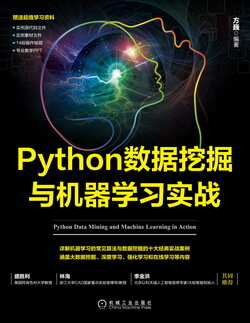# Python 数据挖掘与机器学习实战 (33)：Python 语言简介 2.4(Python 的列表)

list1 = ['a', 'b', 10, 20]list2 = [1, 2, 3, 4]list3 = ["a", "b", "c"]

list1 = ['a', 'b', 10, 20]list2 = [1, 2, 3, 4]print ("list1: ", list1)print ("list2[1:4]: ", list2[1:4])

list1:  alist2[1:4]:  [2, 3, 4]

list = []                                        #空列表list.append('Hello')                             #使用 append() 添加元素list.append('World!')print (list)

['Hello', 'World!']

list1 = ['a', 'b', 10, 20]print (list1)del list1print (" 删除后的输出为 : ")print (list1)

['a', 'b', 10, 20]删除后的输出为 :['a', 'b', 20]

Python 列表操作符和字符串操作符有些是相似的，如“+”号用于组合列表，“*”号用于重复列表。如表 2-3 所示为常见的列表操作符。

len 列表长度
+ 组合
* 重复
in 元素是否存在于列表中
for 迭代

list1 = [1,2,3]list2 = [4,5,6]print(len(list1))print(list1+list2)print(list1*3)print(3 in list1)for x in list1:    print (x)

3[1, 2, 3, 4, 5, 6][1, 2, 3, 1, 2, 3, 1, 2, 3]True123